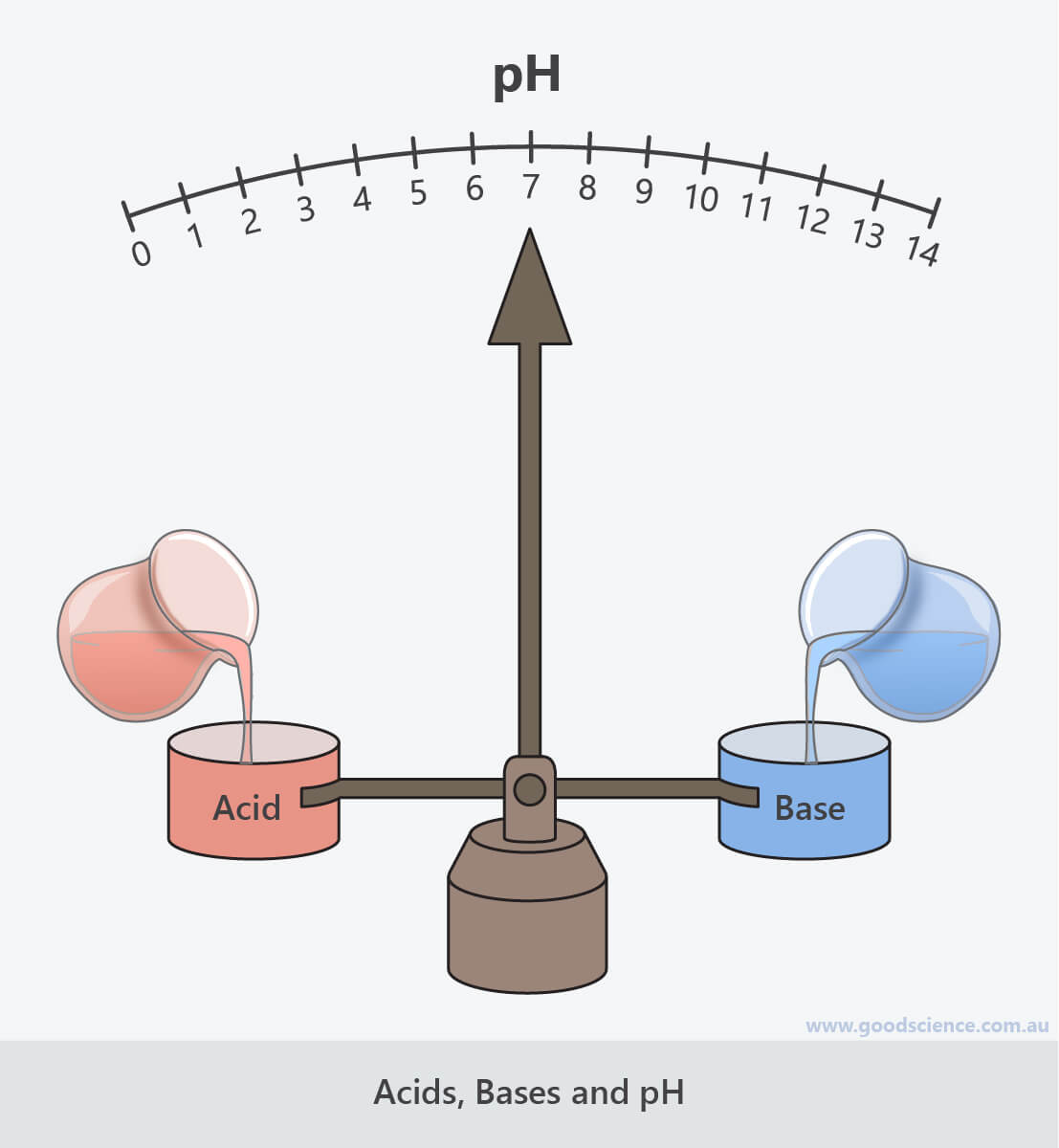Learning Objective

In this lesson we will compare acids, bases and neutral solutions. We will also discuss how acidity is measured and how it can be adjusted.

Learning Outcomes

By the end of this lesson you will be able to:

• Define acids, bases and neutral solutions, in terms of hydrogen ions and hydroxide ions.

• Compare the properties and uses of acids and bases.

• Describe how pH is a measure of the acidity or alkalinity of a solution, and explain how it can be adjusted.

• Describe the use of pH indicators for distinguishing between acids, bases and neutral solutions.

• Distinguish between the terms ‘strong’, ‘weak’, ‘concentrated’ and ‘dilute’, when discussing acids and bases.

BUY THE YEAR 9 CHEMISTRY WORKBOOK

## Introduction

• Substances can be classified as acids, bases or neutral substances.
These vary in their relative concentrations of hydrogen ions and hydroxide ions.
• pH is a measure of how acidic or alkaline (basic) a substance is.
It ranges from 0 (very acidic) to 14 (very alkaline), with 7 being neutral.
• Acids and bases are found widely in nature, and have a variety of scientific and general uses.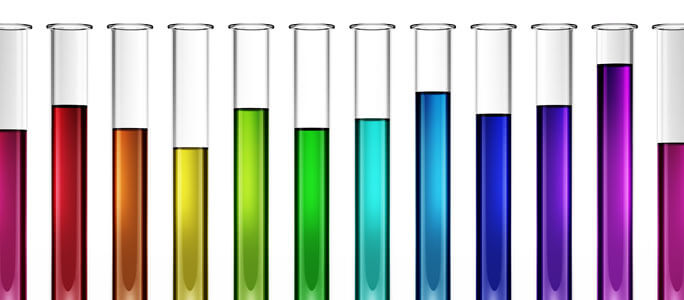## Acids

• Acids are substances that produce hydrogen ions (H+) in solution.
For example, when hydrogen chloride gas is dissolved in water, it ionises to form hydrogen ions and chloride ions, as shown in the following equation:
HCl    H+  +  Cl
• Acids are not only found in laboratories, but also in nature.
For example, acids are found in many foods, including oranges, tomatoes, grapes and milk. Acids are also found in our bodies, and acids are the reason ant bites sting.
• Acids have many uses inside and outside the laboratory.
For example, acids are used in food preservation, car batteries and many industrial chemical processes, to produce substances including plastics, paints and fertilisers.
• Acids taste sour.
For example, think of the taste of lemons or vinegar.
• The chemical names of acids usually end in “acid”.
• Examples of acids commonly used in the laboratory include:
• Hydrochloric acid (HCl).
• Sulfuric acid (H2SO4).
• Nitric acid (HNO3).
• Examples of acids found in nature and foods include:
• Citric acid (oranges, lemons, limes).
• Tartaric acid (grapes, bananas).
• Acetic acid (vinegar).
• Lactic acid (yoghurt, sour cream).
• Formic acid (ant bites, stinging nettle).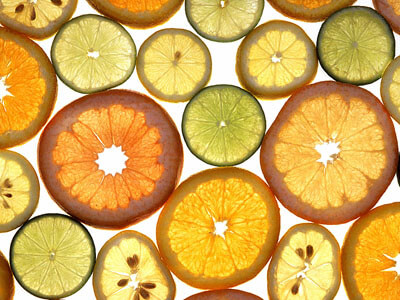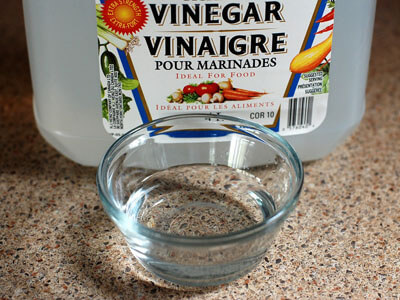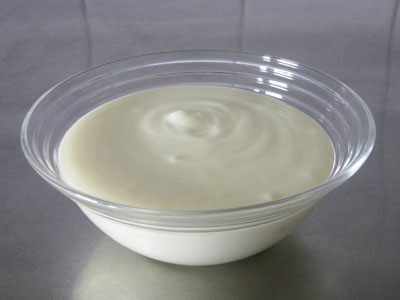Acids are found in many foods, including citrus fruit, vinegar and yoghurt.

(Images: US Department of Agriculture, Wikimedia Commons; evitaochel, Pixabay; pxhere)

## Bases

• Bases are substances that produce hydroxide ions (OH).
For example, when sodium hydroxide is dissolved in water, it dissociates to form sodium ions and hydroxide ions, as shown in the following equation:
NaOH    Na+  +  OH
• Bases that are soluble in water are called alkalis and the solutions they form are called alkaline solutions.
• Their soapy, slimy texture makes bases common components of cleaning products and building materials.
For example, bases are found in a variety of substances, including baking soda, soap, toothpaste, bleach, cement and glass.
• Bases taste bitter.
For example, think of the taste of celery, kale or tonic water.
• The chemical names of bases usually end in “hydroxide”, “oxide”, “carbonate” or “hydrogen carbonate”.
• Examples of common bases, used in and out of laboratories, include:
• Caustic soda (sodium hydroxide – NaOH).
• Chalk, limestone, marble (calcium carbonate – CaCO3).
• Baking soda (sodium bicarbonate – NaHCO3).
• Magnesia (magnesium oxide – MgO).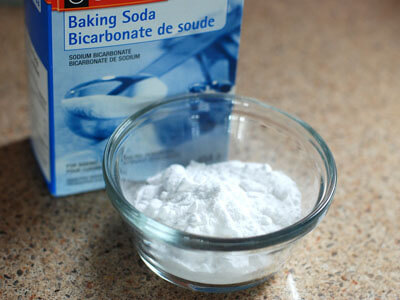Commonly used bases include baking soda, caustic soda and limestone.

(Images: Max Pixel; Skatebiker, Wikimedia Commons; Ferdous, Wikimedia Commons)

## Strong and Weak Acids and Bases

• Acids and bases vary in strength.
Differences in strength relate to the proportion of ions formed.
• When a strong acid or base dissolves in water, all of the molecules form ions.
Example 1
Hydrofluoric acid is a strong acid.
When hydrogen fluoride (HF) dissolves in water, it completely ionises to form hydrogen ions (H+) and chloride ions (Cl).
Example 2
Potassium hydroxide is a strong base.
When potassium hydroxide (KOH) dissolves in water, it completely dissociates to form potassium ions (K+) and hydroxide ions (OH).
• When a weak acid or base dissolves in water, only a small proportion of molecules form ions.
Example 1
Acetic acid is a weak acid.
When acetic acid (CH3COOH) dissolves in water, only about 1% of molecules dissociate to form hydrogen ions (H+) and acetate ions (CH3COO).
Example 2
Ammonia is a weak base*.
When ammonia (NH3) dissolves in water, about 1% of molecules react with water to form ammonium ions (NH4+) and hydroxide ions (OH).
* Weak bases don’t contain hydroxide ions, but instead form hydroxide ions when a small proportion of molecules react with water. Ammonia forms ammonium hydroxide by the following reaction:
NH3  +  H2O    NH4+  +  OH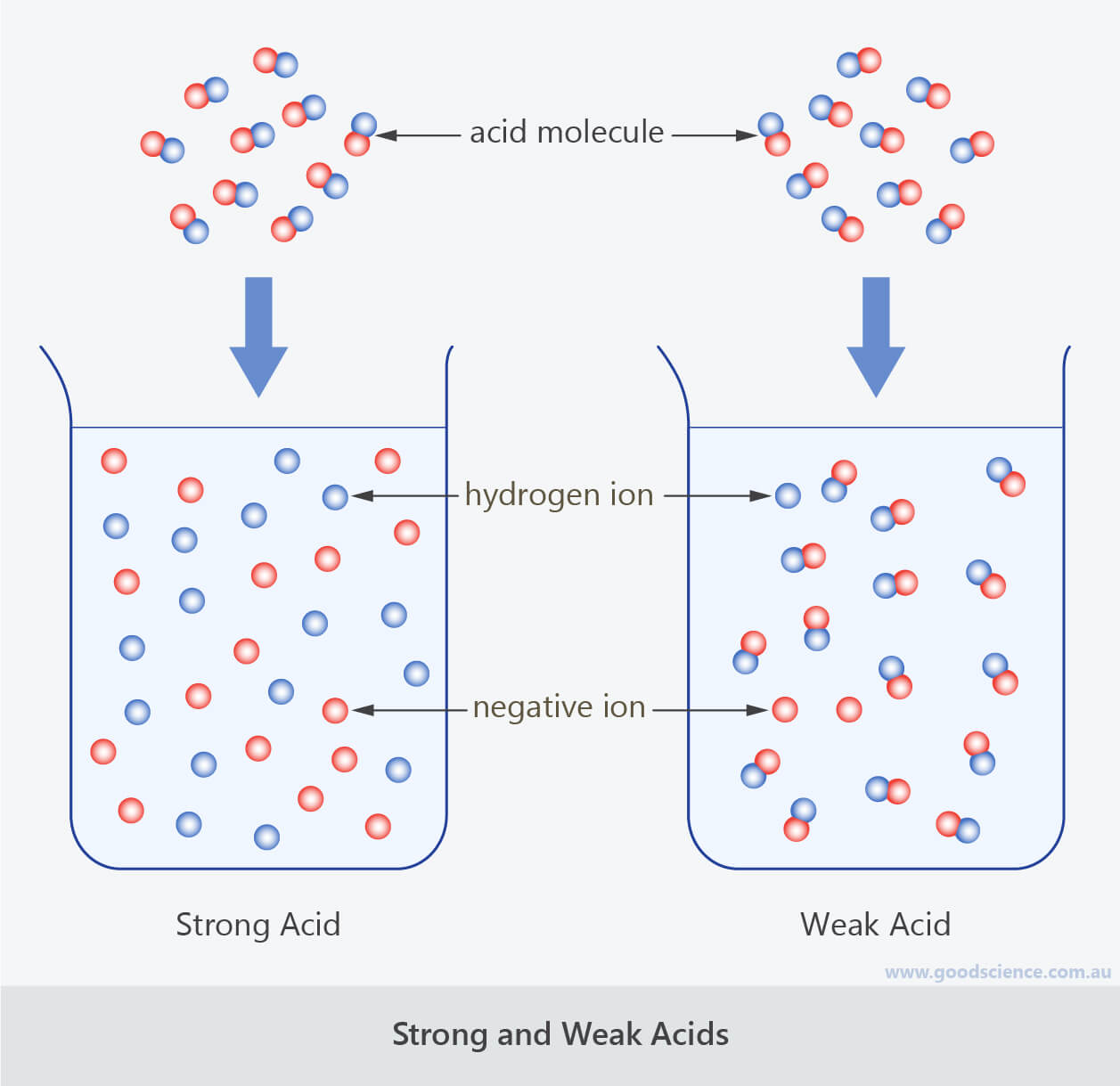Strong acids completely ionise in water.
Weak acids partially ionise in water.

## Concentrated and Dilute Acids and Bases

• A concentrated acid or base is a solution formed from a large number of molecules.
A dilute acid or base is a solution formed from a small number of molecules.
• Note that the definitions of ‘concentrated’ and ‘dilute’ are different to the definitions of ‘strong’ and ‘weak’.
Therefore it is possible for a solution to be:
• A concentrated strong acid or base.
• A dilute strong acid or base.
• A concentrated weak acid or base.
• A dilute weak acid or base.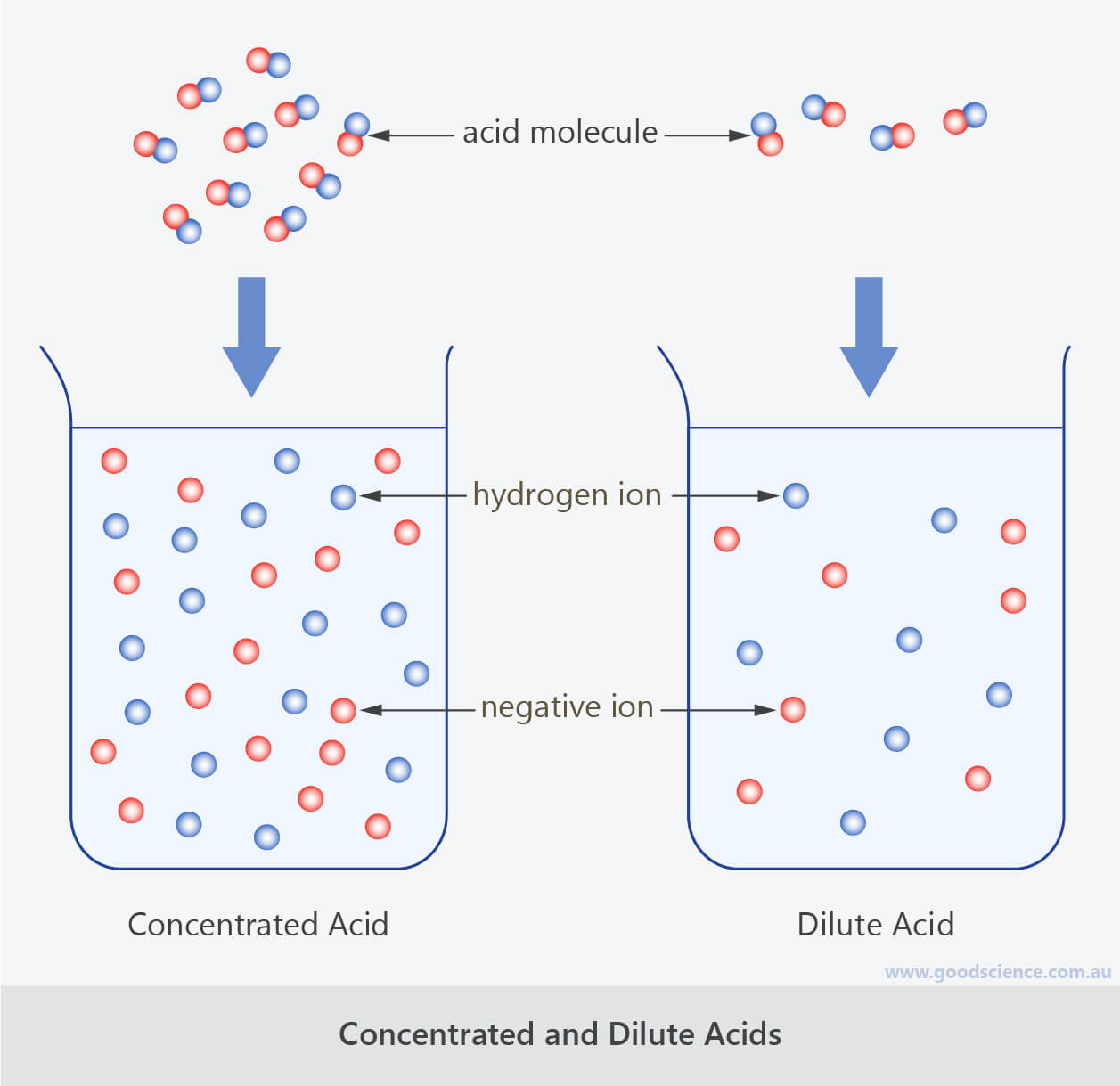Concentrated acids are formed from a large number of molecules.
Dilute acids are formed from a small number of molecules.

## Corrosive Properties of Acids and Bases

• Strong acids and strong bases are both corrosive, which means they react readily with other substances.
For example, acids can cause the corrosion of metals, and alkalis are powerful cleaning agents that can break down grease, oils and fats.
• Strong acids and bases can cause severe burns if they come in contact with living tissue, such as skin or eyes.
Therefore protective clothing, including aprons and safety goggles, should always be worn when handling these materials.
• Even at low concentrations, strong acids and bases are classified as irritants, as they can cause inflammation and reddening of eyes, skin and throat.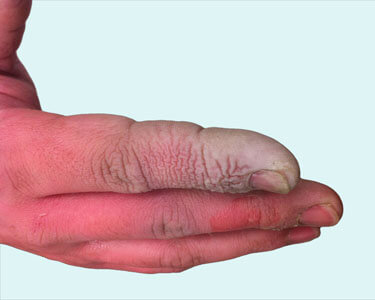Strong acids and bases can cause severe burns, therefore safety gear should always be worn when handling them.

(Images: Dr. Watchorn, Wikimedia Commons; Blazius, Wikimedia Commons; David Dixon, US Air Force)

## The pH Scale

• The pH scale is a measure of how acidic or alkaline a solution is.
• It is usually represented as a numerical scale from 0 to 14.
A solution with a pH less than 7 is acidic.
A solution with a pH greater than 7 is alkaline.
A solution with a pH equal to 7 is neutral – neither acidic nor basic (for example, pure water or salt solution).
• The further away from 7 the pH is, the more acidic or alkaline the solution.
For example, a solution with a pH of 6 is weakly acidic, whereas a solution with a pH of 2 is a strongly acidic.
Similarly, a solution with a pH of 8 is weakly alkaline, whereas a solution with a pH of 12 is a strongly alkaline.
• The pH scale is a logarithmic scale, rather than a linear scale. There is a tenfold increase/decrease in acidity between pH units.
For example, a pH of 5 is ten times more acidic than a pH of 6 and a pH of 9 is ten times more alkaline than a pH of 8.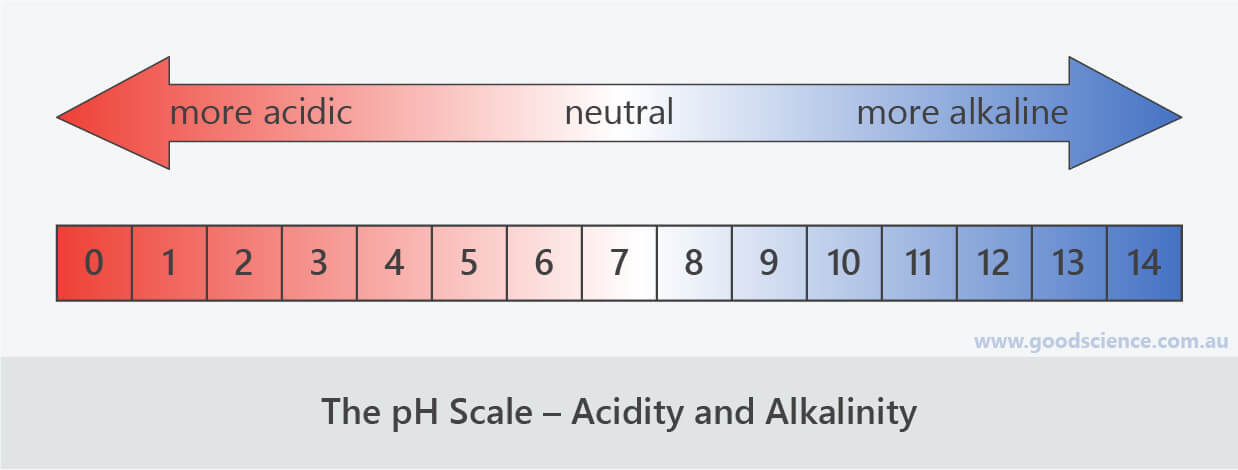The pH scale is a measure of how acidic or alkaline a substance is.

## Concentration of Hydrogen Ions and Hydroxide Ions

• All aqueous solutions (solutions in water) contain hydrogen ions and hydroxide ions.
The pH of a solution is a measure of the relative concentrations of hydrogen ions and hydroxide ions.
• In acidic solutions, the concentration of hydrogen ions is greater than the concentration of hydroxide ions.
In alkaline solutions, the concentration of hydroxide ions is greater than the concentration of hydrogen ions.
In neutral solutions, the concentrations of hydrogen ions and hydroxide ions are equal.
• Since pH is directly related to hydrogen and hydroxide ion concentration, the pH of acids and bases depends on two factors:
1. The strength of the acid or base – the degree to which the molecules form ions in solution.
2. The concentration of the acid or base – how much of the acid or base was added when forming the solution.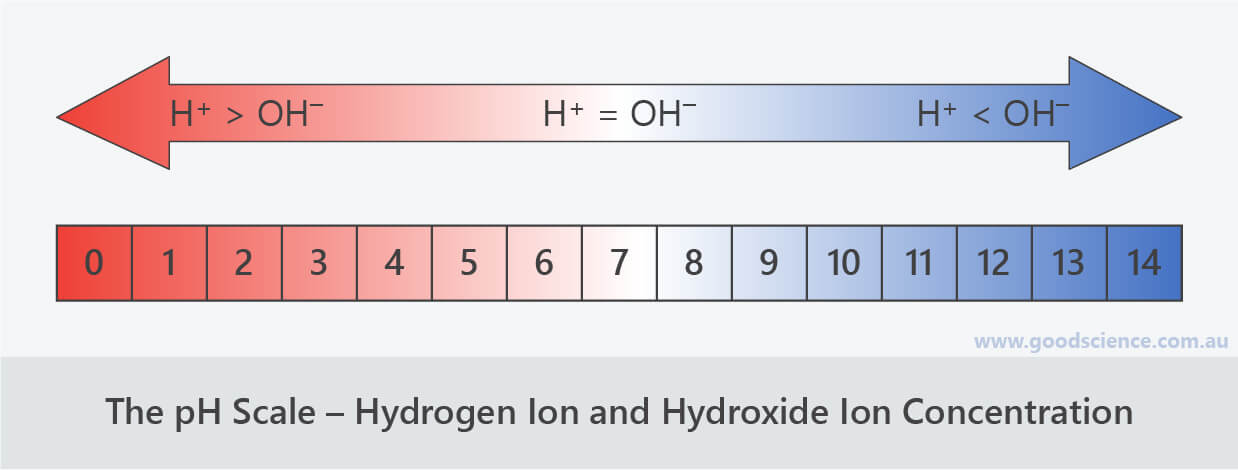The pH scale reflects the relative concentrations of hydrogen ions and hydroxide ions.

## pH Indicators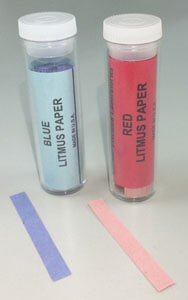• pH indicators are substances that change colour depending on the pH of a solution.
• An example of a pH indicator is litmus paper.
Litmus paper comes in two colours – red and blue. When litmus paper is placed in a solution, it will either change colour or remain the same colour, depending on whether the solution is acidic, alkaline or neutral.
Red litmus paper will turn blue if placed in an alkaline solution, but will remain red if placed in an acidic or neutral solution.
Blue litmus paper will turn red if placed in an acidic solution, but will remain blue if placed in an alkaline or neutral solution.
Two pieces of litmus paper – one red and one blue – are required to distinguish between acidic, alkaline and neutral solutions (see the table below).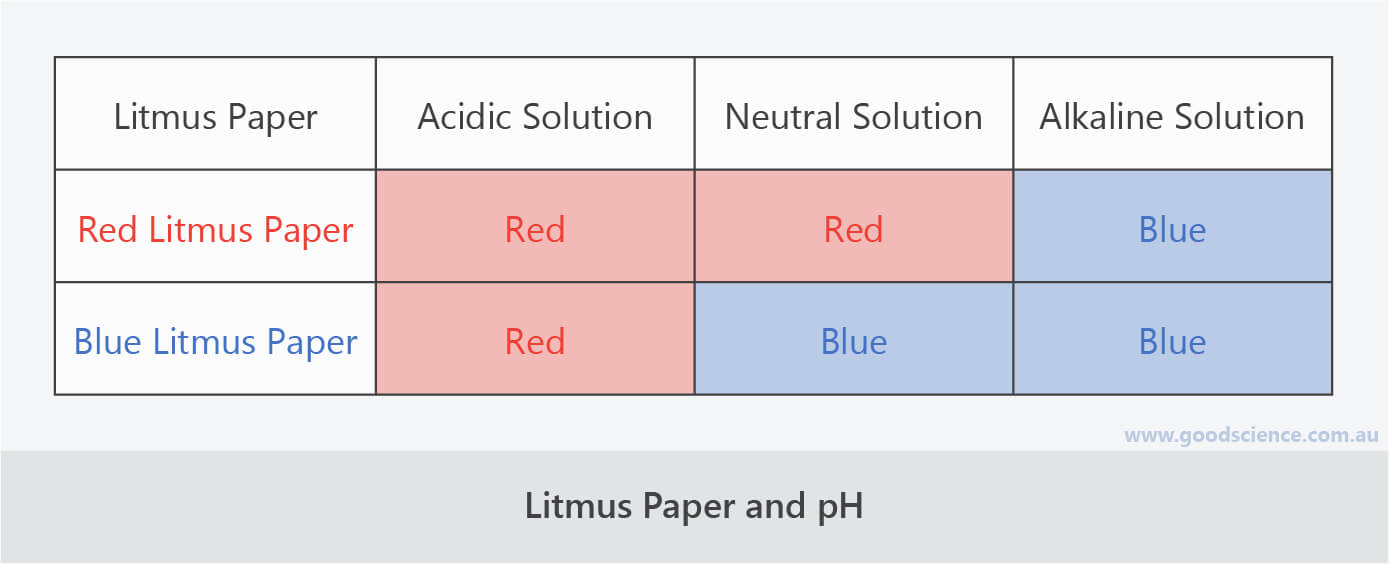Two pieces of litmus paper – one red and one blue – are required to distinguish between acidic, alkaline and neutral solutions.

(Image: Kanesskong, Wikimedia Commons)

• There is a variety of liquid pH indicators which are also used in laboratories.
When 2-3 drops of indicator are added to a small sample of a solution, they cause the solution to change colour, depending on its pH.
Examples of different pH indicators and the colour changes they cause are shown in the table below.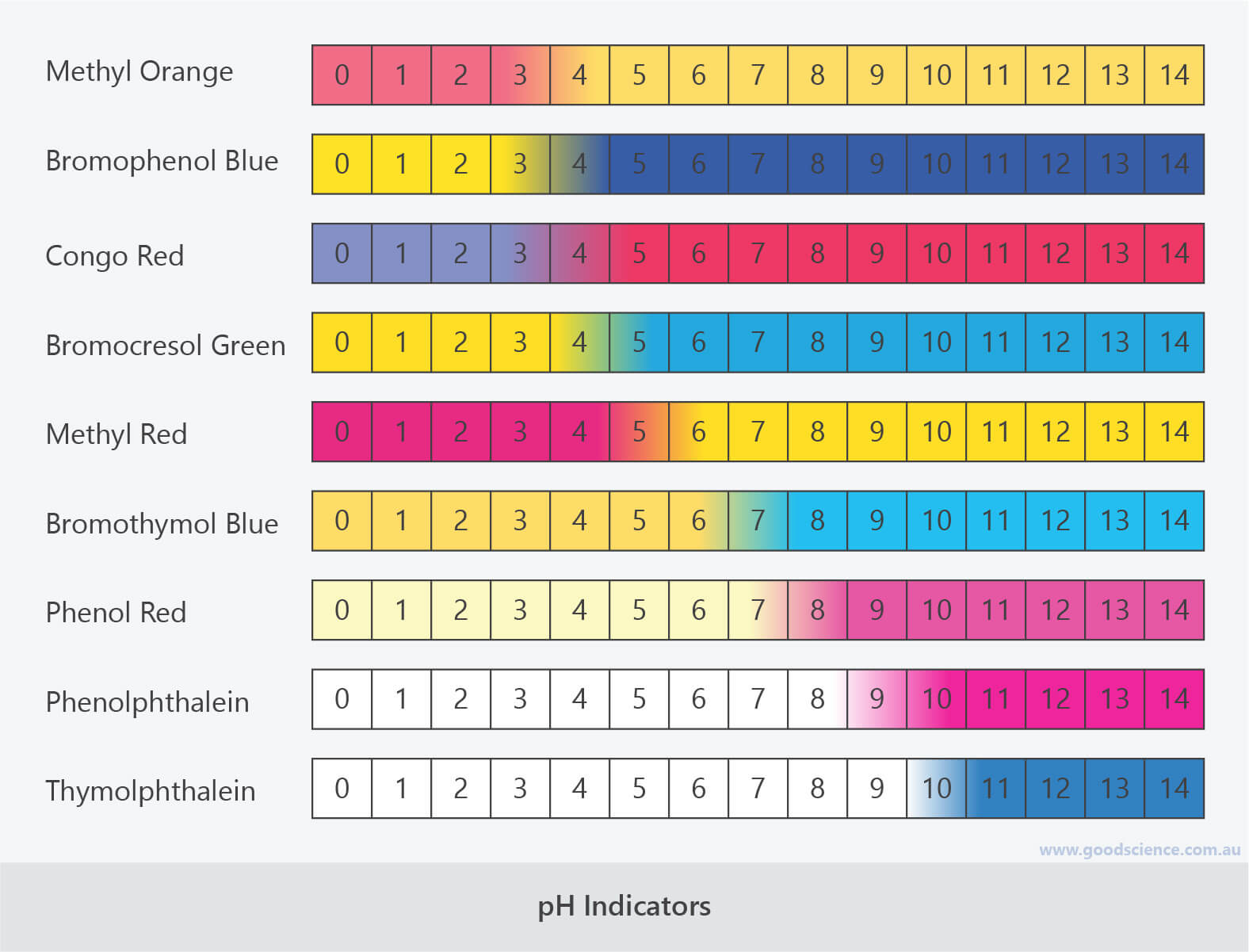pH indicators change colour depending on the pH of a solution.

• Universal indicator, which is actually a mixture of different indicators, produces a range of colours, depending on the pH of a solution.
This makes it especially useful as it can distinguish between strong and weak acids and bases.
Universal indicator also comes with a colour-matching chart, which can be used to determine the approximate pH value of a solution.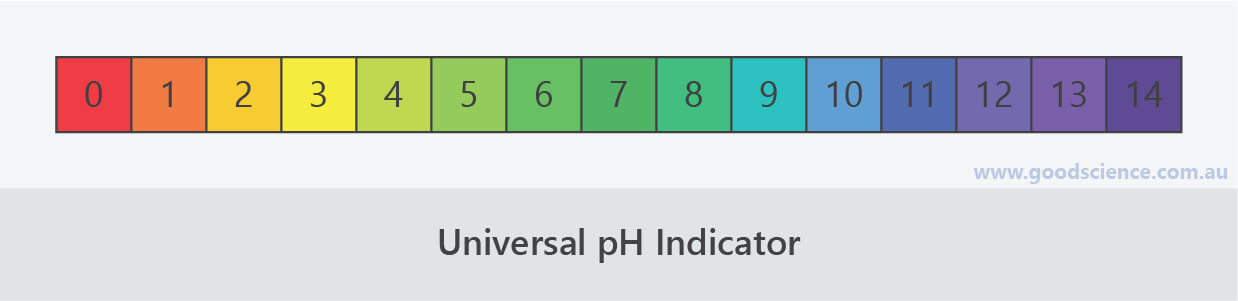Universal pH indicator gives a range of colours for approximating pH value.

• There are many naturally occurring pH indicators which can be extracted from certain fruits, vegetables and garden plants.
These include curry powder, red cabbage, tomatoes, onions, blueberries and grapes.Red cabbage extract, added to solutions across the pH spectrum.

(Image: V. Belkhir, Wikimedia Commons)

• pH meters are electronic scientific instruments that can directly measure hydrogen ion concentration of solutions to give a precise pH value.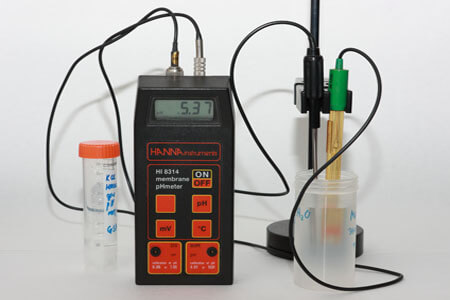pH meters give the most precise measurement of a solution’s pH.

(Image: Sergei Golyshev, Wikimedia Commons)

• The pH of solutions can be adjusted by adding acids or bases to them.
If an acid is added to a solution, the increase in hydrogen ion concentration will cause the pH of the solution to decrease.
If a base is added to a solution, the increase in hydroxide ion concentration will cause the pH of the solution to increase.
• Neutralisation occurs when the pH of an acidic or alkaline solution is adjusted to 7, by adding base or acid respectively, to create a neutral solution with equal amounts of hydrogen ions and hydroxide ions.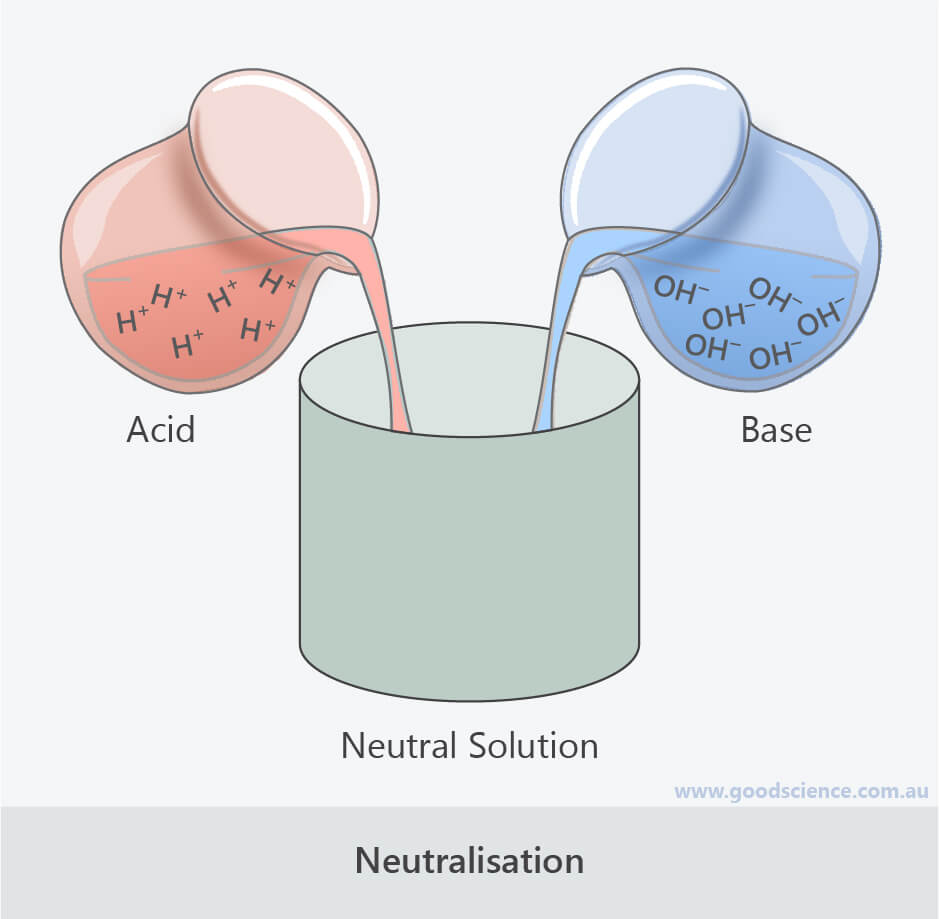Neutralisation occurs when the mixing of acids and bases results in equal numbers of hydrogen ions and hydroxide ions.

• Living organisms are very sensitive to pH. Therefore, careful monitoring and adjusting of pH is necessary in many situations.
For example, swimming pools need to have their pH monitored regularly to ensure they are safe to swim in. If the pH is outside the optimal range of 7.2-7.6, the pool water can cause skin and eye irritation. If the pH needs to be increased, soda ash (sodium carbonate) is used; if the pH needs to be decreased, muriatic acid (hydrochloric acid) is used.
Soil, drinking water, natural waterways and aquariums are other examples where regular pH monitoring is important.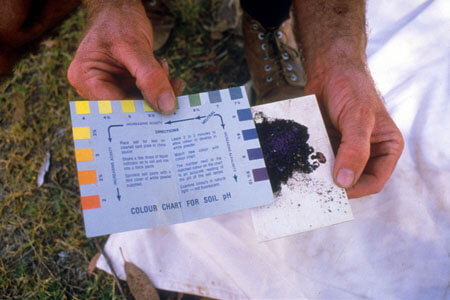Soil pH test kits make it easy for farmers and gardeners to check the pH of their soil.

(Image: CSIRO ScienceImage)

## Summary

• Acids are substances that produce hydrogen ions (H+).
Bases are substances that produce hydroxide ions (OH).
Soluble bases are called alkalis and the solutions they form are called alkaline solutions.
• Acids taste sour, while bases taste bitter.
• Common laboratory acids include hydrochloric acid (HCl), sulfuric acid (H2SO4) and nitric acid (HNO3).
Common laboratory bases include potassium hydroxide (KOH), magnesium oxide (MgO), calcium carbonate (CaCO3) and sodium hydrogen carbonate (NaHCO3).
• A strong acid or base completely ionises in solution.
A weak acid or bases only partially ionises in solution.
• A concentrated acid or base is a solution formed from a large number of molecules.
A dilute acid or base is a solution formed from a small number of molecules.
• The pH scale is a measure of how acidic or alkaline a solution is, based on the relative concentrations of hydrogen ions and hydroxide ions.
It is a numerical scale from 0 to 14.
• Acids have a pH less than 7, and a higher proportion of hydrogen ions than hydroxide ions.
Bases have a pH greater than 7, and a higher proportion of hydroxide ions than hydrogen ions.
Neutral solutions have a pH equal to 7, and an equal proportion of hydrogen ions and hydroxide ions.
• The further away from 7 the pH of a solution is, the greater the difference between hydrogen ion and hydroxide ion concentration, and the more acidic or alkaline the solution.
• The pH of a solution can be increased by adding base.
The pH of a solution can be decreased by adding acid.
Equal amounts of hydrogen ions and hydroxide ions in a solution results in neutralisation.
• pH indicators are substances that change colour depending on the pH of a solution.
• Red litmus paper turns blue if placed in an alkaline solution, but remains red if placed in an acidic or neutral solution.
Blue litmus paper turns red if placed in an acidic solution, but remains blue if placed in an alkaline or neutral solution.
• Universal indicator produces a range of colours, depending on the pH of a solution.
It can distinguish between strong and weak acids and bases, and be used to determine the approximate pH value of a solution.
• pH meters can electronically measure the pH value of a solution.